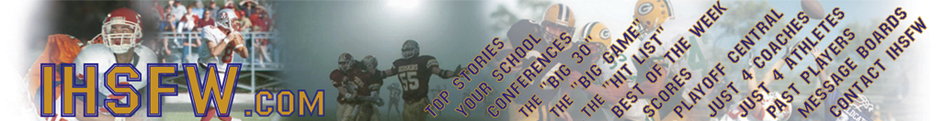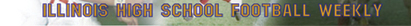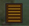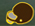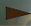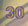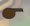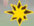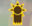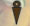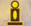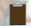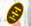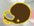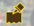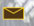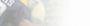Don't see a final on a game you know the score to. E-mail us at IHSFW@IHSFW.com.

2004 Scores

After the end of the regular season, scores will only be posted at Playoff Central.

Week #9
(A-M) / (N-Z)

Week #8
(A-M) / (N-Z)

Week #7
(A-M) / (N-Z)

Week #6
(A-M) / (N-Z)

Week #5
(A-M) / (N-Z)

Week #4
(A-M) / (N-Z)

Week #3
(A-M) / (N-Z)

Week #2
(A-M) / (N-Z)

Week #1
(A-M) / (N-Z)

Scores for the Playoffs will be posted on the Playoff Central page.

Check out Playoff Central for scores, brackets & The Road to Champaign out look.

Scores are collected from games we attend, call-ins and any other methods we can find them by. Not all scores will be displayed right away. If your team isn't on here help us cover them. E-mail scores to scores@ihsfw.com

2003 Scores

2002 Scores

2001 Scores

Week #9
(A-M) / (N-Z)

Week #8
(A-M) / (N-Z)

Week #7
(A-M) / (N-Z)

Week #6
(A-M) / (N-Z)

Week #5
(A-M) / (N-Z)

Week #4
(A-M) / (N-Z)

Week #3
(A-M) / (N-Z)

Week#2

Week #1

2000 Scores

Week #4

A-C / D-L / M-R / S-Z

Week #3
A-C / D-L / M-R / S-Z

Week #2
A-C / D-L / M-R / S-Z